Next: Toomre's Value Up: Gravitational Instability of Rotating Previous: Gravitational Instability of Rotating   Contents

## Tightly Wound Spirals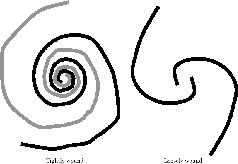We assume the wave driven by the self-gravity has a form of tightly-wound spiral [Fig.3.3(left)]. When we move radially, the density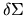varies rapidly. While, it changes its amplitude slowly in the azimuthal direction. In a mathematical expression, if we write the density perturbationas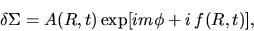(3.21)

where the amplitude of spiral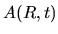is a slowly varing function of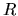, a tightly wound spiral means the shape function varies fast (the radial wavenumber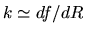is large enough). We consider the gravitational force from the vicinity of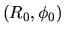, since theoscillates and cancels even if we integrate over large region. Thus,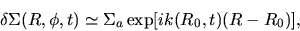(3.22)

where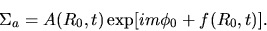(3.23)

Notice that the density perturbation [eq.(3.22)] is similar to that studied in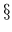2.6. The potential should be expressed in a similar form to equation (2.64) as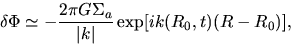(3.24)

which simply means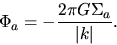(3.25)

If we set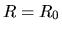, we obtain our final result for the potential due to the surface density perturbation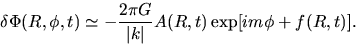(3.26)

Differentiating this equation withand ignoring the term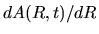compared to that of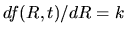, we obtain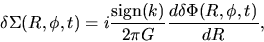(3.27)

Neglecting the terms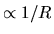compared to the terms containing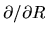, equations (3.18), (3.19), and (3.20) are rewritten as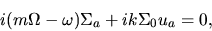(3.28)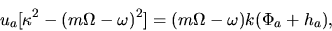(3.29)

and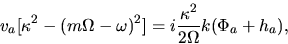(3.30)

Using these equations [(3.28), (3.29), and (3.30)],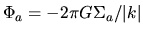, and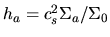, we obtain the dispersion relation for the self-gravitating instability of the rotating gaseous thin disk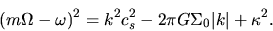(3.31)

Generally speaking, the epicyclic frequency depends on the rotation law but is in the range of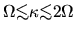(see Table 3.1 for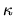for typical rotation laws). It is shown that the system is stabilized due to the epicyclic frequency compared with a nonrotating thin disk [eq.(2.65)].

 RotationRigid-body rotation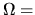const.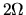Flat rotation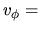const.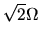Kepler rotation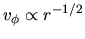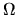Next: Toomre's Value Up: Gravitational Instability of Rotating Previous: Gravitational Instability of Rotating   Contents
Kohji Tomisaka 2007-07-08